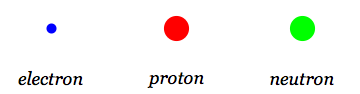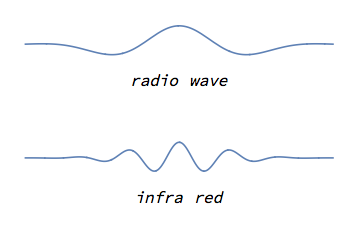## Quantum Mechanics

1.   Everything is a wave
1.1   Particles and waves

### Particles and waves

The entire body of physics developed before quantum mechanics is called classical physics.

In classical physics, people had found two kinds of fundamental entities:

1. Particles: Examples are electrons, protons, neutrons etc. Particles move according to Newton's laws of motion.
2. Waves: Examples are radio waves, infra-red waves etc. The dynamics of these waves is similar to the dynamics of waves on water, and this dynamics is given by a wave-equation.

Thus particles and waves appeared to be very different kinds of entities in classical physics.

But as we will now see, this difference was an illusion.

### The first lesson of quantum mechanics

The main point of quantum theory can be summarized in the following statement:

Everything is a wave.

Radio waves are obviously waves. But if we look carefully, we find that the electron is also a wave.

Why had this fact been missed before? The reason is that the electron wave typically has a very short wavelength. Thus we cannot resolve the up and down motion of the electron wave unless our measuring device is very precise.

As an analogy, consider waves on water. If we look down from an airplane, then a small wave moving on the ocean might look like a fuzzy white ball moving in a straight line. Only when we come close do we notice that this object is actually a wave.

Summary: With quantum theory, we find a beautiful unification: instead of there being two fundamental entities (particles and waves), there is only one fundamental entity: waves. All objects are waves, though in some approximations this wave might look like a moving ball; i.e. a particle.

The next question is: what determines the wavelength of the electron wave, and how small is it?A schematic picture of fundamental particles as imagined in the early twentieth century.Electromagnetic waves with different wavelengths.The electron is also described by a wave.An electron moving in a straight line with constant speed. From a distance this may look like a single blob. But on closer inspection we see that the electron is really described by a wave. The wave can change shape as it evolves; typically it spreads out, as seen here.